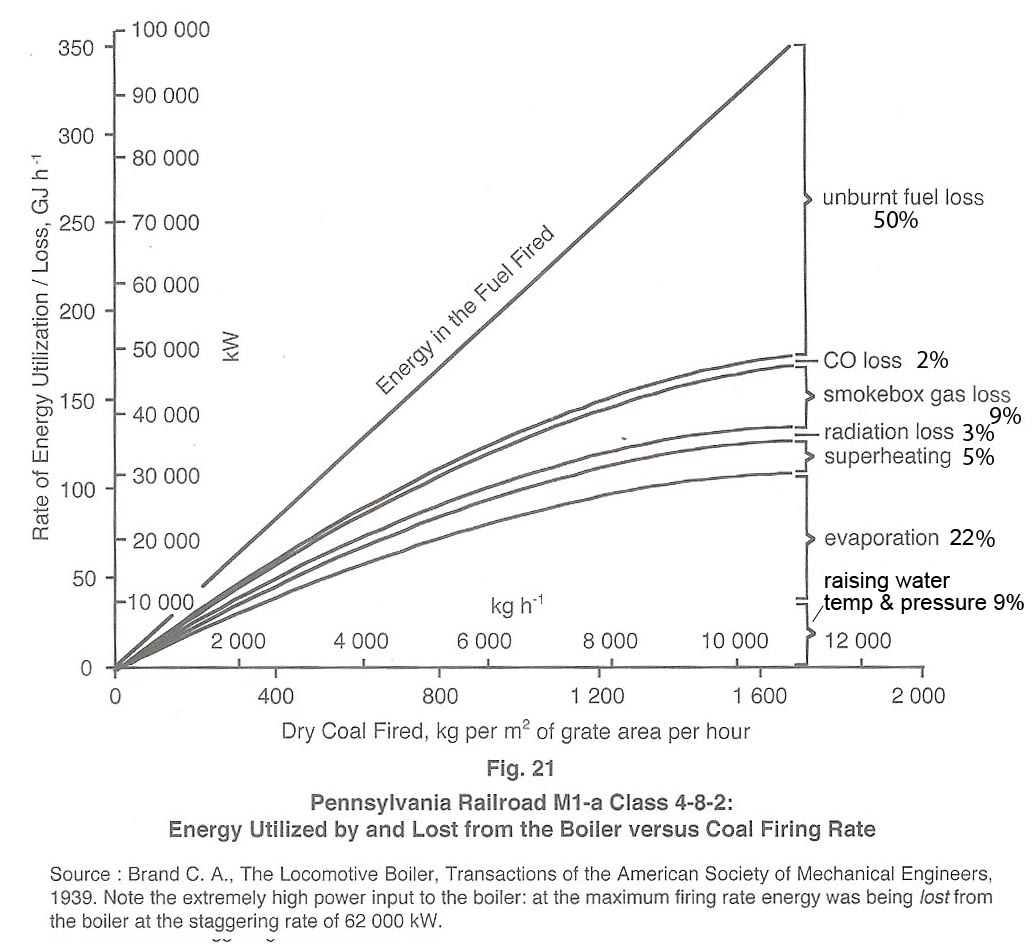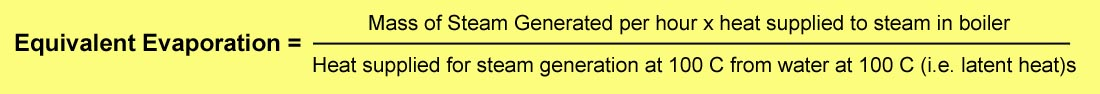# Grate Limit and Boiler Efficiency

## The Grate Limit as it relates to Boiler Efficiency

On page 78 of his book The Red Devil and Other Tales from the Age of Steam, Dave Wardale defines the Grate Limit for a (normal) locomotive firebox as follows:

The grate limit is the point “at which even by firing more coal and supplying more combustion air, no more steam could be produced.”

Put another way, it is the point at which the rate of firing fuel into the firebox exactly equals the rate at which unburned fuel is carried out of the firebox by entrainment in the combustion air.

Wardale quotes an equation derived by L.H. Fry in 1924 that (effectively) relates the grate limit to boiler efficiency as follows:

Eb = k1 – k2 x M/G, where

• Eb = Boiler Efficiency
• M = Firing Rate
• G = Grate Area
• k1 = predicted boiler efficiency at zero firing rate
• k2 = the slope of the graph relating boiler efficiency to firing rate.

The equation is illustrated in graphical form below (Fig 20 in Wardale’s book):The above equation is empirical, yet it is one that produces a fascinating insight – namely that the boiler efficiency at the grate limit is exactly 50% of the predicted efficiency at zero firing rate.

In the diagram, k1 is the maximum predicted boiler efficiency (at zero firing rate) and k2 is the slope of the straight line relating efficiency to firing rate. [A simple mathematical proof that (based on Fry’s equation) the boiler efficiency at the grate limit is exactly half the maximum efficiency is given further below.  A definition of Equivalent Evaporation is also provided at the bottom of the page.]

Wardale goes on to demonstrate that Fry’s equation represents reality, being demonstrated in a boiler test conducted on a Pennsylvania Railroad M1a 4-8-2 locomotive.  Here Wardales adjusts his definition of the grate limit as follows:

The grate limit was the point at which “the heat liberation rate in the firebox was a maximum, which for all practical purposes occurred when the fuel entrained in the draught and escaping unburnt equalled the amount of fuel actually burned, this point being linked to the start of gross firebed fluidisation.”

In other words, the grate limit is reached when half the fuel that is fired into the firebox escapes from the chimney. He illustrates this with the diagram below taken from Fig 21 on page 80 of his book, to which the percentage figures on the right have been added [including an approximate division of “evaporation” into latent and sensible heat]:It should be noted that Fry’s equation does not hold for GPCS fireboxes.  Indeed, the fact that it does not hold is one of the great advantages that GPCS fireboxes offer.

A simple mathematical proof that, based on Fry’s equation, boiler efficiency at the grate limit is exactly half the maximum efficiency, is as follows:

Fry’s Equation:   Eb = k1 – k2 x M/G, where:

• Eb = Boiler Efficiency
• M  = Firing Rate
• G  = Grate Area
• k1 = predicted boiler efficiency at zero firing rate
• k2 = the slope of the graph relating boiler efficiency to firing rate.

Boiler efficiency may also be defined as the amount of energy released from the boiler in the form of steam divided by the amount of energy released in the firebox from the fuel.

Thus if the Steaming Rate = S, then Eb = S ÷ M/G

Thus M/G = S/Eb

Substituting this in Fry’s equation we get:  Eb = k1 – k2 x S/Eb

from which:  Eb2 = k1.Eb – k2.S and thus:  S = k1.Eb/k2 – Eb2/k2

From calculus, we know that S reaches a maximum (or minimum) when the slope of the curve = zero.  This occurs when$\frac { dS }{ d{ E }_{ b } } =\frac { { k }_{ 1 } }{ { k }_{ 2 } } -\frac { 2{ E }_{ b } }{ { k }_{ 2 } } =0$

i.e. when …..${ E }_{ b } = \frac { { k }_{ 1 } }{ 2 }$

Thus the maximum steaming rate occurs at the point where the boiler efficiency is half the predicted value at zero firing rate.

Equivalent Evaporation:

The above graph of Boiler Efficiency vs. Specific Firing Rate includes a curve marked “Equivalent Evaporation”.  Equivalent Evaporation is used as a means of comparing the performance of boilers under a standard set of conditions, and it might be most simply defined as “the quantity of water at 100°C that a boiler can convert into dry/saturated steam at 100°C from each kJ of energy that is applied to it.  This defines it in terms of kg (of water/steam) per kJ of energy.  However it is sometimes defined in units of kg water per kg of fuel, and (as in the case of the graph above) kg water/steam per hour.

A more exact definition of Equivalent Evaporation in units of kg/hr comes from “Thermal Engineering” pages 608/9 by R.K. Rajput (see Google Books):

“Generally the output or evaporative capacity of the boiler is given as kg of water evaporated per hour but as different boilers generate steam at different pressures and temperatures (from feed water at different temperatures) and as such have different amounts of heat ; the number of kg of water evaporated per hour in no way provides the exact means for comparison of the performance of the boilers. Hence to compare the evaporative capacity or performance of different boilers working under different conditions it becomes imperative to provide a common base so that water be supposed to be evaporated under standard conditions. The standard conditions adopted are: Temperature of feed water 100°C and converted into dry and saturated steam at 100°C. As per these standard conditions 1 kg of water at 100°C necessitates 2257 kJ (539 kcal in MKS units) to get converted to steam at 100°C.

“Thus the equivalent evaporation may be defined as: the amount of water evaporated from water at 100°C to dry and saturated steam at 100°C.

“Consider a boiler generating ma kg of steam per hour at a pressure p and temperature T.

Let h = Enthalpy of steam per kg under the generating conditions.

• h = hf + hfg …….   Dry saturated steam at pressure p
• h = hf + xhfg …….   Wet steam with dryness fraction x at pressure p
• h = hf + hfg + cp (Tsup – Ts) ….. Superheated steam at pressure p and temperature Tsup
• hf1 = Specific enthalpy of water at a given feed temperature.

Then heat gained by the steam from the boiler per unit time  =  ma x (h – hf1)

The equivalent evaporation (me) from the definition is obtained as:$\frac { dS }{ d{ E }_{ b } } =\frac { { k }_{ 1 } }{ { k }_{ 2 } } -\frac { 2{ E }_{ b } }{ { k }_{ 2 } } =0$

The evaporation rate of the boiler is also sometimes given in terms of kg of steam /kg of fuel. The presently accepted standard of expressing the capacity of a boiler is in terms of the total heat added per hour.

An alternative definition is offered by Applied Thermodynamics by Onkar Singh as follows:

“For comparing the capacity of boilers working at different pressures, temperatures, different final steam conditions etc, a parameter called “equivalent evaporation” can be used.  Equivalent evaporation actually indicates the amount of heat added in the boiler for steam generation.  Equivalent evaporation refers to the quantity of dry saturated steam generated per unit of time from feedwater at 100°C to steam at 100°C at the saturation pressure corresponding to 100°C. Sometimes it is called equivalent evaporation from and at 100°C.  Thus mathematically it could be given as:For a boiler generating steam at ‘m’ kg/h at some pressure ‘p’ and temperature ‘T’, the heat supplied for steam generation =  m x (h – hw), where h is the enthalpy of final steam generated and hw is enthalpy of feedwater.  Enthalpy of final steam shall be:

• h = hf + hfg = hg for final steam being dry saturated steam (hf, hfg and hg are used for their usual meanings),
• h = hf + x . hfg for wet steam as final steam,
• h = hg + cp sup.steam . (Tsup – Tsat) for superheated final steam.

Equivalent evaporation (kg/kg of fuel) =$\frac { m.(h-{ h }_{ w }) }{ 539 }$

Equivalent evaporation is thus a parameter which could be used for comparing the capacities of different boilers.”

Note – the last equation purports to express equivalent evaporation in units of kg/kg of fuel, but in fact the units are actually in kg/hr.]